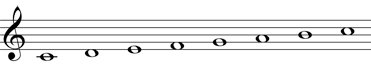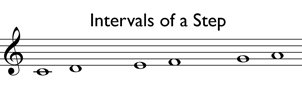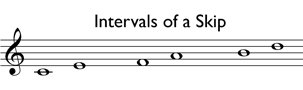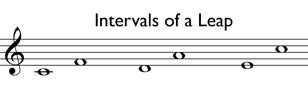# General Intervals: Step, Skip, and Leap

One way to describe and characterize a melody is by analyzing its intervals. An interval is the distance between two pitches. A melodic interval refers specifically to the distance between two notes that are played one at a time.

In the most general terms, melodic intervals can be described as moving by step, skip, and leap.

A step is the distance from one pitch to the next pitch in a diatonic scale. Observe the C diatonic scale below and use it as a reference for determining the intervals.As shown in the example below, the interval from C to D is a step. The distance from E to F is a step, and so is the interval between G and A.An interval of a skip is equal to the distance of two steps. In other words, the pitches “skip” over the interval of a step. For example, the distance from C to E is a skip. The distance from F to A is a skip, and so is the interval between B and D.Any interval larger than a skip is called a leap. Therefore, the leap can be pretty wide. The interval from C to F is a leap. The distance from D to A is a leap, and so is E to C.Intervals can be defined much more specifically than the general terms of step, skip, and leap, as shown in the following lessons. However, when learning to perform a melody, it is good to understand and recognize these basic intervals.